RRB JE CE (CBT I) Mock Test- 9

# RRB JE CE (CBT I) Mock Test- 9 - Civil Engineering (CE)

Test Description

## 100 Questions MCQ Test RRB JE Mock Test Series for Civil Engineering (CE) - RRB JE CE (CBT I) Mock Test- 9

RRB JE CE (CBT I) Mock Test- 9 for Civil Engineering (CE) 2023 is part of RRB JE Mock Test Series for Civil Engineering (CE) preparation. The RRB JE CE (CBT I) Mock Test- 9 questions and answers have been prepared according to the Civil Engineering (CE) exam syllabus.The RRB JE CE (CBT I) Mock Test- 9 MCQs are made for Civil Engineering (CE) 2023 Exam. Find important definitions, questions, notes, meanings, examples, exercises, MCQs and online tests for RRB JE CE (CBT I) Mock Test- 9 below.
Solutions of RRB JE CE (CBT I) Mock Test- 9 questions in English are available as part of our RRB JE Mock Test Series for Civil Engineering (CE) for Civil Engineering (CE) & RRB JE CE (CBT I) Mock Test- 9 solutions in Hindi for RRB JE Mock Test Series for Civil Engineering (CE) course. Download more important topics, notes, lectures and mock test series for Civil Engineering (CE) Exam by signing up for free. Attempt RRB JE CE (CBT I) Mock Test- 9 | 100 questions in 90 minutes | Mock test for Civil Engineering (CE) preparation | Free important questions MCQ to study RRB JE Mock Test Series for Civil Engineering (CE) for Civil Engineering (CE) Exam | Download free PDF with solutions
 1 Crore+ students have signed up on EduRev. Have you?
RRB JE CE (CBT I) Mock Test- 9 - Question 1

### Three taps A, B, and C can fill a tank in 20, 24, and 30 hours respectively. How long (in hours) would the three taps take to fill the tank if all of them are opened together?

Detailed Solution for RRB JE CE (CBT I) Mock Test- 9 - Question 1
A can fill 1 unit of water in the tank in 1/20 hours

B can fill 1 unit of water in the tank in 1/24 hours

C can fill 1 unit of water in tank in 1/30 hours

(A+B+C) can fill 1 unit of water in tank in 1/20+1/24+1/30=6+5+4/120 =15/120=18 days

Therefore,

(A+B+C) can fill a full tank in 8 days.

RRB JE CE (CBT I) Mock Test- 9 - Question 2

### If the rate of interest is 8% per annum and Rs. 10000 lent at the compound interest half yearly then calculate the equivalent simple rate of interest for the first year?

Detailed Solution for RRB JE CE (CBT I) Mock Test- 9 - Question 2
Let the principal be Rs.100

the rate of interest is 8% per annum lent at the compound interest half yearly.

Compound interest for 6 months = 4%

Compound interest next 6 months = 4%+4of 4%=(4+.16)%=4.16%

the equivalent simple rate of interest for the first year=4+4.16=8.16%

RRB JE CE (CBT I) Mock Test- 9 - Question 3

### PAB and PCD are two secants to a circle. if PA =10cm , AB =12cm and PC =11cm, then what is the value of PD?

Detailed Solution for RRB JE CE (CBT I) Mock Test- 9 - Question 3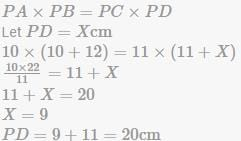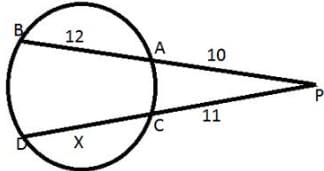RRB JE CE (CBT I) Mock Test- 9 - Question 4

The speed of a boat in still water is 10 km/hr. It covers a distance of 50 km upstream in 6 hours. What is the speed (in km/hr) of the stream?

Detailed Solution for RRB JE CE (CBT I) Mock Test- 9 - Question 4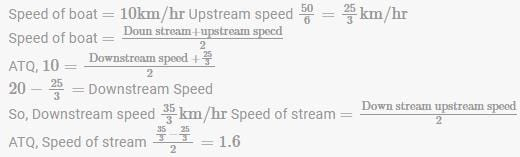RRB JE CE (CBT I) Mock Test- 9 - Question 5

500 litres of milk solution has 40% milk in it. How much more milk should be added to make it 60% in the solution?

Detailed Solution for RRB JE CE (CBT I) Mock Test- 9 - Question 5
Milk = 40% of 500 = 200 litres

Water is 60% of 500 = 300 litres

ATQ,

40%of mixture=water=300 litres

New mixture quantity=300/40% =7 50 liters

The quantity of milk in the mixture= 60 % of 750=450 liters

Required milk to be mixed=450-200=250 liters

Therefore,

250 liter of milk should be added to make it 60% in the solution.

RRB JE CE (CBT I) Mock Test- 9 - Question 6

The shadow of a tower on ground level is increased by 20 m, when the angle of depression by the sun changes from 60 to 30. The height of the tower is?

Detailed Solution for RRB JE CE (CBT I) Mock Test- 9 - Question 6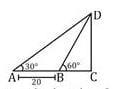Let the height of tower DC = h meter tan⁡60=h/BC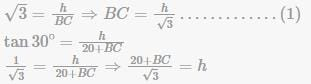Putting the value of BC from equation(1) in equation (2), we get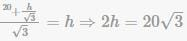∴ h=10√3 meter

RRB JE CE (CBT I) Mock Test- 9 - Question 7

What will be the sum of all odd whole numbers between 10 and 38?

Detailed Solution for RRB JE CE (CBT I) Mock Test- 9 - Question 7
To calculate sum, we need to find number of odd whole numbers between 10 and 38 using the Arithmetic Progression formula.

That is,

Last term = First term + (number of terms-1) × Common difference

ATQ,

37 = 11 + (N-1) × 2

So, N or Number of terms =14

Now,

Sum of terms = N/2 [2×first term(N−1)×d]

ATQ,

Sum = 14/2 [2×11+(14−1)×2]

Sum = 336

Therefore,

The Sum of all odd whole numbers between 10 and 38 is 336

RRB JE CE (CBT I) Mock Test- 9 - Question 8

If the volume of two cubes is in the ratio 125 : 1, then what is the ratio of their edges?

Detailed Solution for RRB JE CE (CBT I) Mock Test- 9 - Question 8
volume of cube1 =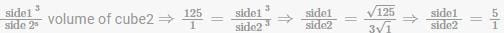RRB JE CE (CBT I) Mock Test- 9 - Question 9

A ,B and C invest in a business in the ratio 3:6:5. A and C are working partners.Only B is a sleeping partner hence his share will be 3/4th of what it would have been if he were a working partner. If they make Rs. 50000 profit, half of which is reinvested in the business and the other half is distributed between partners, then how much does C get (in Rs )?

Detailed Solution for RRB JE CE (CBT I) Mock Test- 9 - Question 9
Given the investment ratio

old ratio

A:B:C

3: 6: 5

ATQ. B′ s share =6×(3/4)=9/2

new ratio 3:9/2:5

6: 9: 10

total profit =50000 after reinvestment the profit =50000/2=25000

C's share = (25000x10)/25 = Rs. 10000

RRB JE CE (CBT I) Mock Test- 9 - Question 10

The HCF and LCM of two numbers are 45 and 270 respectively. If the ratio of the two numbers is 2:3 and smaller of the two numbers is X, then find X2

Detailed Solution for RRB JE CE (CBT I) Mock Test- 9 - Question 10
We know that

Product of numbers =product of lcm and hcf

2y×3y=45×270

y2 = 45×45

y=45

then smaller no. X=2×45=90

X2 = (90)2=8100

RRB JE CE (CBT I) Mock Test- 9 - Question 11

In how many years will Rs 2,000 yield Rs 662 as compound interest at 10% per annum compounded annually?

Detailed Solution for RRB JE CE (CBT I) Mock Test- 9 - Question 11
Compound interest in 1 year compounded quarterly = Then, rate =(R)% per year time =(n) quarters, = P x (1 + (R/100))n - P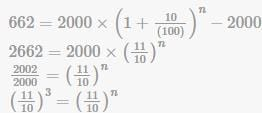n = 3 years

RRB JE CE (CBT I) Mock Test- 9 - Question 12

What is the value of tan (π/4 + A) × tan (3π/4 + A)?

Detailed Solution for RRB JE CE (CBT I) Mock Test- 9 - Question 12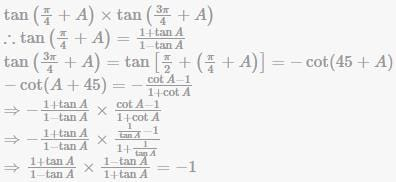RRB JE CE (CBT I) Mock Test- 9 - Question 13

The distance between the centers of the two circles is 61cm and their radius is 35cm and 24cm. What is the length(in cm) of the direct common tangent to the circles?

Detailed Solution for RRB JE CE (CBT I) Mock Test- 9 - Question 13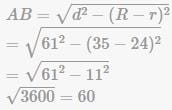RRB JE CE (CBT I) Mock Test- 9 - Question 14

What is the value of 2cot P−A/2/1+tan2 2P−A/2 ?

Detailed Solution for RRB JE CE (CBT I) Mock Test- 9 - Question 14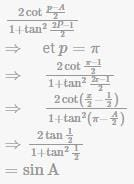RRB JE CE (CBT I) Mock Test- 9 - Question 15

Find the standard deviation of {11, 7, 10, 13, 9}

Detailed Solution for RRB JE CE (CBT I) Mock Test- 9 - Question 15
First we have to find the mean Mean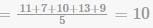Standard deviation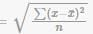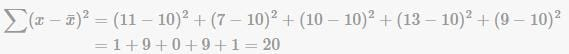Standard deviation =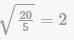RRB JE CE (CBT I) Mock Test- 9 - Question 16

If a dairy mixes cow’s milk which contains 10%fat with buffalo’s milk which contains 20% fat,then the resulting mixture has the fat of 120/7% . In what ratio was the cow’s milk mixed with buffalo’s milk?

Detailed Solution for RRB JE CE (CBT I) Mock Test- 9 - Question 16
use alligation rule,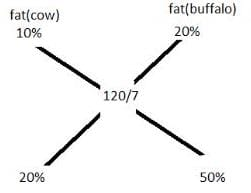ratio 2:5

RRB JE CE (CBT I) Mock Test- 9 - Question 17

If two complimentary angles are in the ratio of 4:5, find the greater angle.

Detailed Solution for RRB JE CE (CBT I) Mock Test- 9 - Question 17
Let the angles be 4x , 5x

4x+5x=90

9x=90

x=10

Greater angle = 5x=50

RRB JE CE (CBT I) Mock Test- 9 - Question 18

If cosθ = 4/5, then (secθ+cosecθ)=

Detailed Solution for RRB JE CE (CBT I) Mock Test- 9 - Question 18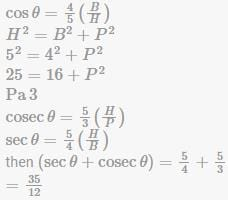RRB JE CE (CBT I) Mock Test- 9 - Question 19

In the given figure, in a right angle triangle ABC, AB=12 cm and AC =15cm. A square is inscribed in the triangle. One of the vertices of the square coincides with the vertex of the triangle. What is the maximum possible area of the square ( cm2 )?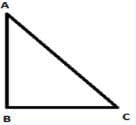Detailed Solution for RRB JE CE (CBT I) Mock Test- 9 - Question 19
AB=12cm,AC=15cm

then, BC=152−122=9cm

side of square =(P×B)/(P+B) where, Pe perpendicular, B = base side of the square =(12×9)/21=36/7

area of the largest square that can be formed inside the triangle = (36/7)2 = (1296/49)y

RRB JE CE (CBT I) Mock Test- 9 - Question 20

What is the measure of the two equal angles of a right isosceles triangle?

Detailed Solution for RRB JE CE (CBT I) Mock Test- 9 - Question 20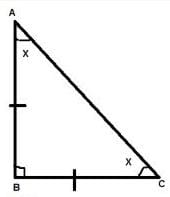A.T.Q ∠B=90

and AB=BC

So, ∠A=∠C

we know that ∠A+∠B+∠C=180

x+90+x=180

2x=90

X = 450

RRB JE CE (CBT I) Mock Test- 9 - Question 21

If 1082 =11664 , then what is the value of √1.1664 + √116.64 ?

Detailed Solution for RRB JE CE (CBT I) Mock Test- 9 - Question 21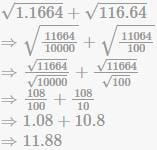RRB JE CE (CBT I) Mock Test- 9 - Question 22

The length and breadth of a rectangular field are in the ratio of 5 : 2. If the perimeter of the field is 140 metres, then what is the breadth (in metres)?

Detailed Solution for RRB JE CE (CBT I) Mock Test- 9 - Question 22
Let the Length and Breadth of the rectangle are 5x and 2x respectively.

140=2(5x+2x)

70=7x

So, x=10 m

Therefore, Breadth of rectangle = 2×10=20 metre

RRB JE CE (CBT I) Mock Test- 9 - Question 23

Calculate the amount on Rs. 37,500 @ 8% p.a compounded half yearly for 1 1/2 years.

Detailed Solution for RRB JE CE (CBT I) Mock Test- 9 - Question 23
As the interest compounded half yearly So time will be =3 half year Rate =8/2=4%

amount =P(1+(R/100))n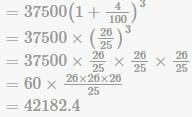RRB JE CE (CBT I) Mock Test- 9 - Question 24

The mean of 17 numbers is 12. If two numbers 9 and 15 are removed, then what will be the mean of the remaining number?

Detailed Solution for RRB JE CE (CBT I) Mock Test- 9 - Question 24
Sum of 17 numbers = 17 ×12= 204

After removal of 9 and 15, the the sum becomes 180.

Therefore,

Mean of remaining 15 numbers = 180/15=12

RRB JE CE (CBT I) Mock Test- 9 - Question 25

Ram Naresh got two successive discounts, a bag with a list price of Rs. 400 is available at Rs. 160. If the second discount is 20%, find the first discount.

Detailed Solution for RRB JE CE (CBT I) Mock Test- 9 - Question 25
Let the first discount be x%

First a discount of 20% means that you multiply the price by .80(1−0.20) The initial price is therefore first multiplied by x, then by 0.80, and the result is 160 . 400×x×0.80=160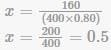Multiply by 0.5 means reduce by 50%

RRB JE CE (CBT I) Mock Test- 9 - Question 26

If the diagonal of a square is 12 cm, then what is the area (in cm2 ) of the square?

Detailed Solution for RRB JE CE (CBT I) Mock Test- 9 - Question 26
Diagonal of square =side⁡ x√2

Area of square =sid⁡e2

ATQ, Diagonal =12cm Side ×√2=12

So, Side =12√2 Therefore, Area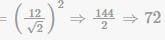RRB JE CE (CBT I) Mock Test- 9 - Question 27

A line passing through (3, 5) makes angle of 135 with x-axis. What will be its equation?

Detailed Solution for RRB JE CE (CBT I) Mock Test- 9 - Question 27
Slope=tan 1350 = −1=m

⇒ A (3,5)

(y-5)=-(x-3)

Equation of line

x+y-8=0

RRB JE CE (CBT I) Mock Test- 9 - Question 28

Three toys are in the shape of a cylinder, hemisphere, and cone. The three toys have the same base. The height of each toy is 2 √2. What is the ratio of the total surface areas of the cylinder, hemisphere, and cone, respectively?

Detailed Solution for RRB JE CE (CBT I) Mock Test- 9 - Question 28
Height of each toy =2√2 since the height of hemisphere = radius of the hemisphere

= radius of each toy =2√2 slant height of cone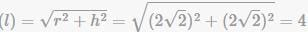ratio = T.S.A of cylinder : T.S.A of hemisphere : T.S.A of cone =2πr(h+r):3πr2:πr(l+r)

=2(h+r):3r:(l+r)

=2(4√2):3×2√2:(4+2√2)

= 4 : 3 : √2 + 1

RRB JE CE (CBT I) Mock Test- 9 - Question 29

A sum of Rs 4000 becomes Rs 5800 in 3 years, when invested in a scheme of simple interest. If the same sum is invested in a scheme of compound interest with the ame yearly interest rate (compounding of interest is done yearly), then what will be the amount (in Rs) after 2 years?

Detailed Solution for RRB JE CE (CBT I) Mock Test- 9 - Question 29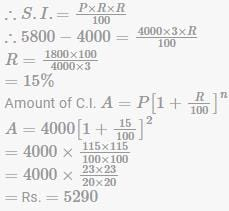RRB JE CE (CBT I) Mock Test- 9 - Question 30

Average of the runs of 133 players of a team is 38. If the average of the runs of the male players is 43 and the average of the runs of the female players is 24, then what will be the ratio of the total runs of male players and the total runs of female players respectively?

Detailed Solution for RRB JE CE (CBT I) Mock Test- 9 - Question 30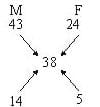M:F=14:10=14:5

No. of males

=(14/19)×133=98

Total runs of males

=98×43

No. of females

=133−98=35

Total runs of females

=35×24

Required ratio = (98 x 43)/(35 x 24) = 301/60

RRB JE CE (CBT I) Mock Test- 9 - Question 31

Argument and which is a 'weak' argument and choose the corresponding option as your answer.

Should religion be taught in schools in a secular state?

I. No, because in a secular state, indoctrination of children would be wrongful.

II. Yes, religion helps in inculcating moral values in a child.

Detailed Solution for RRB JE CE (CBT I) Mock Test- 9 - Question 31
I is a weak argument as it is taking about the indoctrination of children. In a secular state, all religions are given equal status, and choosing to teach or learn any religion should be allowed. 'Indoctrination' is a strong word to be used to define the ,kmkmkmkm religion. II is a strong argument, as it gives a benefit of teaching religion. B is the right answer.
RRB JE CE (CBT I) Mock Test- 9 - Question 32

Identify the diagram that best represents the relationship among the given classes.

Toys, plastic, wood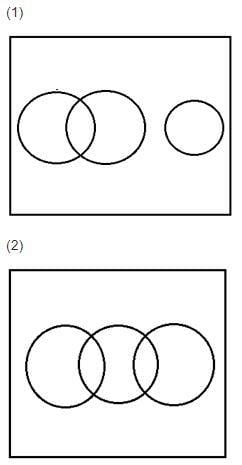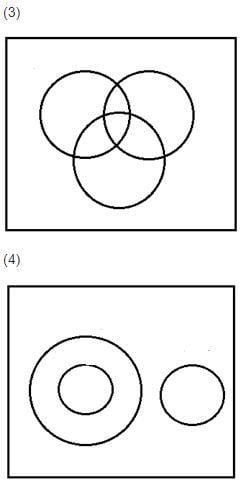Detailed Solution for RRB JE CE (CBT I) Mock Test- 9 - Question 32
Some toys are of wood and some toys are of plastic. No plastic is wood
RRB JE CE (CBT I) Mock Test- 9 - Question 33

Three years ago, the average age of a family of 5 members was 17 years. The current average age of the family is equal to the average age of the family 3 years ago, due to the birth of a child. What is the present age of the child?

Detailed Solution for RRB JE CE (CBT I) Mock Test- 9 - Question 33
Total age of the family 3 years ago = 17 x 5 = 85 years

Total age of the family now = 17 x 6 = 102 years

Total age of the family excluding the child now = 85 + 15 = 100 years

Age of the child = 2 years

RRB JE CE (CBT I) Mock Test- 9 - Question 34

Seven people A, B, C, D, E, F, G were standing in a row according to their age in decreasing order such that the eldest person was standing in the front. Further it is known that G who was elder to F was standing adjacent to A. C was second youngest and was standing just behind E. F was not the youngest while D was standing at the front. A was not standing adjacent to D. How many people were standing between A and B?

Detailed Solution for RRB JE CE (CBT I) Mock Test- 9 - Question 34
D was standing at the front, so D was the eldest. C was second youngest and was standing just behind E, so E was third youngest.

D > ___ >___>___> E > C> ___

Now G was elder to F and adjacent to A. Also A was not seated adjacent to D.

D > G > A > ___>E > C >___

Since F was not the youngest. Hence

D > G > A > F > E > C > B.

So three people were seated between A and B.

RRB JE CE (CBT I) Mock Test- 9 - Question 35

If AMPHIBIOUS is written as MAHPBIOISU then how will ANTIBIOTIC be written as?

Detailed Solution for RRB JE CE (CBT I) Mock Test- 9 - Question 35
The word is divided into 5 groups of 2 letters. The letters in each group is written in the reverse order. Thus, ANTIBIOTIC will be written as NAITIBTOCI
RRB JE CE (CBT I) Mock Test- 9 - Question 36

Which of the following will be the mirror image of the given question figure, if a mirror is placed along the line MN.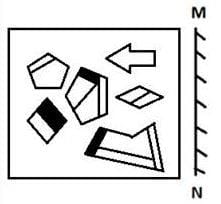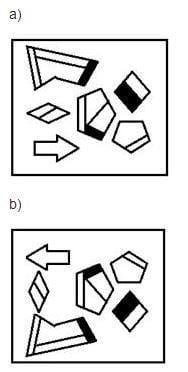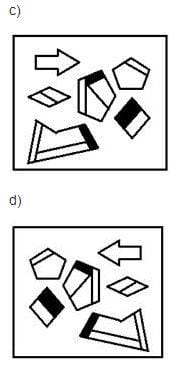Detailed Solution for RRB JE CE (CBT I) Mock Test- 9 - Question 36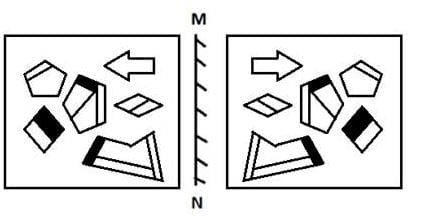RRB JE CE (CBT I) Mock Test- 9 - Question 37

In each of the questions below, an assertion (a) is given, followed by a reason (R) which may or may not explain the assertion. From the given options, choose the right answer.

A: When we chew rice for a long time, it begins to taste sweet.

R: Ptyalin, an enzyme contained in saliva, breaks down starch into glucose.

Detailed Solution for RRB JE CE (CBT I) Mock Test- 9 - Question 37
R is the correct reason for A, as this enzyme breaks down starch, which is contained in rice, into glucose which leads to its sweet taste. (A) is the right answer.
RRB JE CE (CBT I) Mock Test- 9 - Question 38

A boy started walking straight for 5 kms. Then he took a right turn and walked for 2 kms. Then he again turned right and walked for 10 kms. Then he turned to his left and walked for 3 kms. It was the time for sunset and he observed that his shadow falls to his left. In which direction was he facing when he started walking from the starting point?

Detailed Solution for RRB JE CE (CBT I) Mock Test- 9 - Question 38
Al sunset, the shadow talks to the east. We have the path traversed by the boy as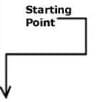RRB JE CE (CBT I) Mock Test- 9 - Question 39

A two-digit number is 20% more than the number, which is the reverse of that number. What is the number?

Detailed Solution for RRB JE CE (CBT I) Mock Test- 9 - Question 39
Let the number be 10a+b

The reverse number is 10b+a

10a+b= (10b+a) [1+(20/100)]

10a+b= (10b+a) [6/5]

50a+5b= 60b+6a

44a= 55b

4a= 5b

a/b = 5/4

Hence the number is 10a+b = 10x5+4 = 54

RRB JE CE (CBT I) Mock Test- 9 - Question 40

F is the brother of A. C is the daughter of A. K is the sister of F, G is the brother of C. who is the uncle of G ?

Detailed Solution for RRB JE CE (CBT I) Mock Test- 9 - Question 40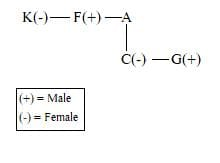RRB JE CE (CBT I) Mock Test- 9 - Question 41

Mark the correct option based on which statement/statements are sufficient to answer the question.

How many sisters does Ramya have?

I. Antara is the sister of Ramya and is the youngest in the family

II. Ramya who is Sita's sister, has two siblings

Detailed Solution for RRB JE CE (CBT I) Mock Test- 9 - Question 41

From I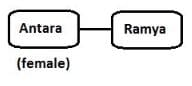So statement I only is not sufficient to answer the question.

From II.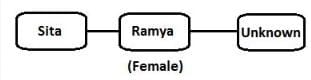So statement II only is not sufficient to answer the question.

From 1 and 11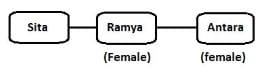So we cannot answer how many sisters Ramya has because we do not know the gender of Sila.

Please note in reasoning questions one cannot assume the genders of a the the person even if they have names that are very commonly used for a male or female.

So, option d is the correct answer.

RRB JE CE (CBT I) Mock Test- 9 - Question 42

In each of the questions below, two statements are given, followed by two conclusions. You have to consider the given statements as true, even if they are at variance from commonly known facts. From the given options, choose the one that provides the correct combination of conclusions that follow/ can be derived from the given statements.

Statement A: Vineyards flourish, and wine is made in all parts of the kingdom.

Statement B: But that wine which is imported so largely into England, is made only from the grapes grown on the steep hillsides, as they are the finest of the lot.

Conclusions:

I: Only the wine made from the finest grapes is imported largely into England from the kingdom.

II: Wine is made only from grapes grown on steep hillsides.

Detailed Solution for RRB JE CE (CBT I) Mock Test- 9 - Question 42
Statement B mentions that the grapes grown on steep hillsides are the finest of the lot, which is why they are used to make the wine which is imported into England. So, this confirms I. II is incorrect, as the statements together imply that wine is made in all parts of the kingdom, not only on steep hillsides. A is the right answer, as only I follows.
RRB JE CE (CBT I) Mock Test- 9 - Question 43

Find the odd one.

Detailed Solution for RRB JE CE (CBT I) Mock Test- 9 - Question 43
68 + 31 = 99

73 + 31 = 104

54 + 31 = 85

63 + 21 = 84

RRB JE CE (CBT I) Mock Test- 9 - Question 44

In each of the following questions, select a figure from amongst the four alternatives, which when placed in the blank space of figure (X) would complete the pattern.

Identify the figure that completes the pattern.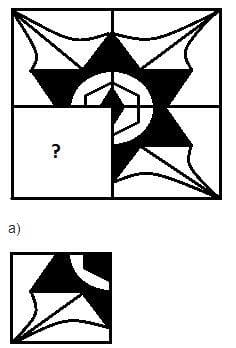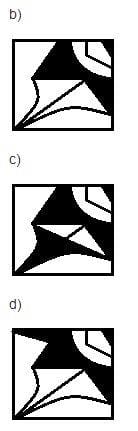Detailed Solution for RRB JE CE (CBT I) Mock Test- 9 - Question 44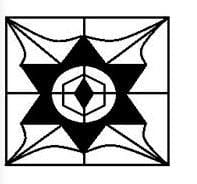RRB JE CE (CBT I) Mock Test- 9 - Question 45

In the following question, which one set of letters when sequentially placed at the gaps in the given letter series shall complete it?

b _ d e _ b c _ e f _ c _ _ f b _ d _ f

Detailed Solution for RRB JE CE (CBT I) Mock Test- 9 - Question 45
b c d e f / b c d e f / b c d e f / b c d e f
RRB JE CE (CBT I) Mock Test- 9 - Question 46

A is the father of C and D is son of B. E is brother of A. If C is sister of D, how is B related to E?

Detailed Solution for RRB JE CE (CBT I) Mock Test- 9 - Question 46
We get the following family tree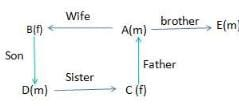Clearly B is the sister - in - law of E.

RRB JE CE (CBT I) Mock Test- 9 - Question 47

In the following question, four figures marked A, B, C and D, are given. Among these, three figures follow the same pattern and remaining one figure doesn't follow that pattern and hence, is odd. Mark the option which denotes the odd figure.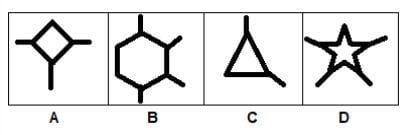Detailed Solution for RRB JE CE (CBT I) Mock Test- 9 - Question 47
Figure B is odd as, rest others have number of lines which drawn from the vertices are one less than the number of vertices of respective diagram. Figure B have 6 vertices with 4 lines drawn from the vertices.
RRB JE CE (CBT I) Mock Test- 9 - Question 48

In each of the questions below are given some statements followed by two conclusions numbered I and II. You have to take the given statements to be true even if they seem to be at variance from commonly known facts.

Read all the conclusions and then decide which of the given conclusions logically follows from the given statements disregarding commonly known facts.

Statements:

Some petals are corals.

No coral is a deal.

No petal is a steel.

Conclusions:

I. No deal is a coral.

II. Some deals are steels.

Detailed Solution for RRB JE CE (CBT I) Mock Test- 9 - Question 48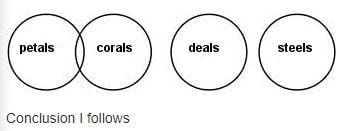RRB JE CE (CBT I) Mock Test- 9 - Question 49

How many triangles are present in the given figure?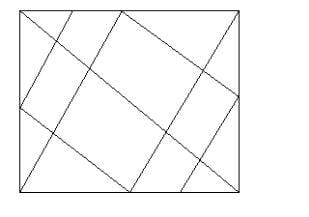Detailed Solution for RRB JE CE (CBT I) Mock Test- 9 - Question 49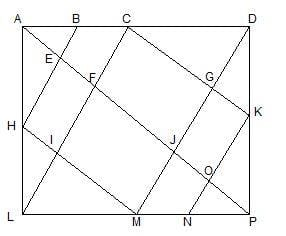On one side of diagonal AP triangles are- ABE, ACF, ADJ, ADP, CDG, CDK, DGK, DJP, KOP = 9 triangles

So, on both sides = 2x9= 18 triangles.

But, apart from these 18, we have-

ABH, ACL, PNK, PMD = 4 triangles.

Thus, we have total triangles = 18+4= 22 triangles in the given figure.

RRB JE CE (CBT I) Mock Test- 9 - Question 50

Select the answer figure from the given alternatives, which can be formed by folding the given question figure?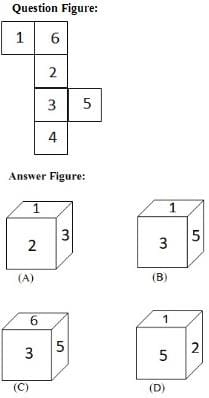Detailed Solution for RRB JE CE (CBT I) Mock Test- 9 - Question 50
After folding the figure

6 lies opposite 3.

2lies opposite 4

1 lies opposite 5

1 and 5 cannot be on the adjacent faces.

6 and 3 cannot be on the adjacent faces.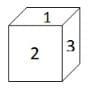RRB JE CE (CBT I) Mock Test- 9 - Question 51

A class consists of 180 students, 50 % passed in Maths, 10% students failed in Maths and English and 20% students passed in both Maths and English. Find the number of students passed only in English

Detailed Solution for RRB JE CE (CBT I) Mock Test- 9 - Question 51
Total students = 180

Failed in both = 10% of 180

=> 18

=> Passed in both = 20% of 180

=> 36

=> Passed in maths = 50% 0f 180

=> 90

=> Passed only in math = 90-36

=> 54

=> Passed only in English = 180 - (54 + 36 + 18)

=> 72

RRB JE CE (CBT I) Mock Test- 9 - Question 52

A plece of paper is folded and cut as shown in the figure: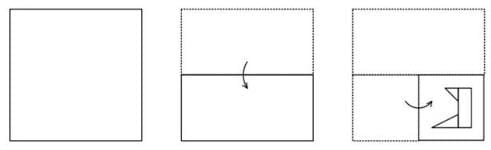How will it appear when it is unfolded?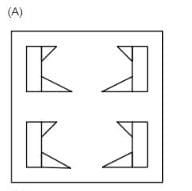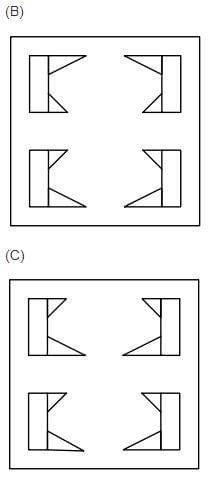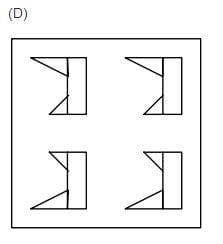Detailed Solution for RRB JE CE (CBT I) Mock Test- 9 - Question 52
After 1st unfolding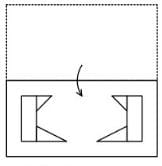After 2nd unfolding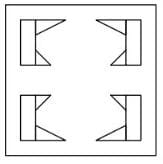So, option 2 is the correct answer.

RRB JE CE (CBT I) Mock Test- 9 - Question 53

The series is given, with one term missing. Choose amongst the given responses and complete the series.

AZP, CWR, ETT, GQV, __.

Detailed Solution for RRB JE CE (CBT I) Mock Test- 9 - Question 53
1st letter of each term is shown by a line from A to F.

2nd letter of each term is shown by a line from Z to N.

3rd letter of each term shown by the dotted line from P to X.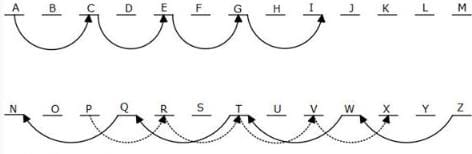So INX is the correct answer.

RRB JE CE (CBT I) Mock Test- 9 - Question 54

If A is directly proportional to the square of B, B is inversely proportional to the square of C, and C is directly proportional to D, then what will be the change in the value of A if the value of D is increased by 200%?

Detailed Solution for RRB JE CE (CBT I) Mock Test- 9 - Question 54
As per the given information in the question

C is directly proportional to D

D increases by 200% so it becomes 3 times

So, C becomes 3 times.

B is directly proportional (1/C2) = 1/9

B becomes (1/9) times

A is directly proportional B2 = (1/9)2 = (1/81) times

A becomes (1/81) times.

RRB JE CE (CBT I) Mock Test- 9 - Question 55

In each of the following questions, you are given a figure (X) followed by four alternative figures (a), (b), (c) and (d) such that figure (X) is embedded in one of them. Trace out the alternative figure which contains fig. (X) as its part.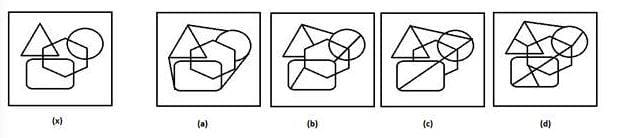Detailed Solution for RRB JE CE (CBT I) Mock Test- 9 - Question 55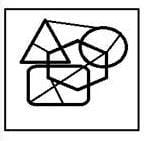RRB JE CE (CBT I) Mock Test- 9 - Question 56

When the East India Company was formed, the Mughal emperor in India was_____ .

Detailed Solution for RRB JE CE (CBT I) Mock Test- 9 - Question 56
The English East India Company was founded in 1600. Akbar was Mughal Emperor from 1556 until his death in 1605.
RRB JE CE (CBT I) Mock Test- 9 - Question 57

Buland Darwaza was built by -

Detailed Solution for RRB JE CE (CBT I) Mock Test- 9 - Question 57
Buland Darwaza was built by Akbar.A stunning monument that signifies courage and victory, the Buland Darwaza is a 17th-century imperial gate located in Fatehpur Sikri, Agra. The word Buland means “Victorious.” This gate was constructed to commemorate the Mughal Emperor Akbar's victory over the kingdom of Gujarat in 1573.
RRB JE CE (CBT I) Mock Test- 9 - Question 58

Which one of the following countries shares the shortest boundary with India?

Detailed Solution for RRB JE CE (CBT I) Mock Test- 9 - Question 58
Myanmar. shares the shortest boundary with India.
RRB JE CE (CBT I) Mock Test- 9 - Question 59

Who is the current Governor of Haryana?

Detailed Solution for RRB JE CE (CBT I) Mock Test- 9 - Question 59
Satyadev Narayan Arya is the current Governor of Haryana.
RRB JE CE (CBT I) Mock Test- 9 - Question 60

The DRDO was established in:

Detailed Solution for RRB JE CE (CBT I) Mock Test- 9 - Question 60
The DRDO was established in 1958 with just 10 laboratories to enhance the research work in defence sector.
RRB JE CE (CBT I) Mock Test- 9 - Question 61

The maximum canal irrigated area is in the state of:

Detailed Solution for RRB JE CE (CBT I) Mock Test- 9 - Question 61
The maximum canal irrigated area is in the Uttar Pradesh.
RRB JE CE (CBT I) Mock Test- 9 - Question 62

The Bhakra Nangal dam is built on the river?

Detailed Solution for RRB JE CE (CBT I) Mock Test- 9 - Question 62
Bhakra Dam is built on the river Satluj in Bilaspur, Himachal Pradesh, northern India.
RRB JE CE (CBT I) Mock Test- 9 - Question 63

The first meeting of India and _______________ Joint Committee on Border Haats was held in Agartala recently.

Detailed Solution for RRB JE CE (CBT I) Mock Test- 9 - Question 63
The first meeting of the India and Bangladesh Joint Committee on Border Haats was held in Agartala recently.
RRB JE CE (CBT I) Mock Test- 9 - Question 64

Third Battle of Panipat was fought between–

Detailed Solution for RRB JE CE (CBT I) Mock Test- 9 - Question 64
The third Battle of Panipat was fought between Ahmed Shah Abdali and the Marathas
RRB JE CE (CBT I) Mock Test- 9 - Question 65

‘Dudhwa National Park’ is situated in-

Detailed Solution for RRB JE CE (CBT I) Mock Test- 9 - Question 65
‘Dudhwa National Park’ is situated in Uttar Pradesh.
RRB JE CE (CBT I) Mock Test- 9 - Question 66

Part III of the Constitution of India relates to?

Detailed Solution for RRB JE CE (CBT I) Mock Test- 9 - Question 66
(a) Fundamental Rights are mentioned in part III of the Indian Constitution.
RRB JE CE (CBT I) Mock Test- 9 - Question 67

By which name/names is our country mentioned in the Constitution?

Detailed Solution for RRB JE CE (CBT I) Mock Test- 9 - Question 67
(a) The constitution states: India, also known as Bharat, is a Union of States. It is a Sovereign Socialist Secular Democratic Republic with a parliamentary system of government. The Republic is governed in terms of the Constitution of India which was adopted by the Constituent Assembly on 26th November, 1949 and came into force on 26th January, 1950. The Constitution provides for a Parliamentary form of government which is federal in structure with certain unitary features. The constitutional head of the Executive of the Union is the President.
RRB JE CE (CBT I) Mock Test- 9 - Question 68

Under the Ayushman Bharat Yojana, Prime Minister Narendra Modi has inaugurated the first Health and Wellness Centre in -

Detailed Solution for RRB JE CE (CBT I) Mock Test- 9 - Question 68
Prime Minister Narendra Modi inaugurated the first Health and Wellness Centre in Bijapur, Chhattisgarh to mark the launch of Ayushman Bharat Yojana- National Health Protection Mission (AB-NHPM).
RRB JE CE (CBT I) Mock Test- 9 - Question 69

Who has been appointed as Finance Secretary?

Detailed Solution for RRB JE CE (CBT I) Mock Test- 9 - Question 69
Shri A. N. Jha appointed as Finance Secretary.
RRB JE CE (CBT I) Mock Test- 9 - Question 70

Who founded the Mughal dynasty in India?

Detailed Solution for RRB JE CE (CBT I) Mock Test- 9 - Question 70
RRB JE CE (CBT I) Mock Test- 9 - Question 71

Which process is used in order to make ghee ?

Detailed Solution for RRB JE CE (CBT I) Mock Test- 9 - Question 71
Hydrogenation is the process of adding hydrogen to an edible vegetable oil using a catalyst to produce fat with semi solid.
RRB JE CE (CBT I) Mock Test- 9 - Question 72

Which of the following is called ring disease in plants?

Detailed Solution for RRB JE CE (CBT I) Mock Test- 9 - Question 72
Wilt of potato is also known as ring disease because the brown ring is formed on the Xylem of the plant. The factor of this is Pseudomonas solanacearum bacteria. In this disease, the conduction system of the plant is also affected.
RRB JE CE (CBT I) Mock Test- 9 - Question 73

The density of the gas is maximum at which of the following factors?

Detailed Solution for RRB JE CE (CBT I) Mock Test- 9 - Question 73
The density of the gas is maximum at Low temperature and high pressure. A The density, or more precisely, the volumetric mass density, of a substance is its mass per unit volume. The symbol most often used for density is ρ, although the Latin letter D can also be used.
RRB JE CE (CBT I) Mock Test- 9 - Question 74

Which of the following animals do belong to the mollusca phylum?

Detailed Solution for RRB JE CE (CBT I) Mock Test- 9 - Question 74
Octopus belongs to the phylum Mollusca. Mollusca is a large phylum of invertebrate animals whose members are known as molluscs or mollusks. Around 85,000 extant species of molluscs are recognized.
RRB JE CE (CBT I) Mock Test- 9 - Question 75

Which of the following gene does induce cancer?

Detailed Solution for RRB JE CE (CBT I) Mock Test- 9 - Question 75
Cancer is induced by Oncogene. An oncogene is a gene that has the potential to cause cancer. In tumor cells, they are often mutated and/or expressed at high levels. Most normal cells will undergo a programmed form of rapid cell death (apoptosis) when critical functions are altered and malfunctioning.
RRB JE CE (CBT I) Mock Test- 9 - Question 76

The following component of a fluorescent lamp contributes maximum in making them costly is______?

Detailed Solution for RRB JE CE (CBT I) Mock Test- 9 - Question 76
In a fluorescent lighting system, the ballast regulates the current to the lamps and provides sufficient voltage to start the lamps. Without a ballast to limit its current, a fluorescent lamp connected directly to a high voltage power source would rapidly and uncontrollably increase its current draw.
RRB JE CE (CBT I) Mock Test- 9 - Question 77

What is a Carbamide?

Detailed Solution for RRB JE CE (CBT I) Mock Test- 9 - Question 77
Ceramide is another name of Urea, an organic compound with chemical formula CO(NH2)2 . This name indicates that Urea is an amide in which two – NH2 groups are joined by a carbonyl (C=O) functional group.
RRB JE CE (CBT I) Mock Test- 9 - Question 78

Na+ The number of electrons in is-

Detailed Solution for RRB JE CE (CBT I) Mock Test- 9 - Question 78
The sodium atom has 11 protons, 11 electrons and 12 neutrons. On the other hand Na+ has 11 protons, 10 electrons and 12 neutrons. An Na+ ion is a sodium atom that has lost one electron as that makes the number of electrons in the atom equal to that of the nearest Nobel gas Neon which has 10 electrons.
RRB JE CE (CBT I) Mock Test- 9 - Question 79

The particular element that is used in preparing windows for X-Ray Tubes is_________?

Detailed Solution for RRB JE CE (CBT I) Mock Test- 9 - Question 79
The particular element that is used in preparing windows for X-Ray Tubes is Beryllium
RRB JE CE (CBT I) Mock Test- 9 - Question 80

Which among the following is the first phase in the sexual reproduction of organisms?

Detailed Solution for RRB JE CE (CBT I) Mock Test- 9 - Question 80
Gametogenesis is the process by which diploid precursor cells undergo meiotic division to become haploid gametes (sex cells) In males, this process is called spermatogenesis and produce spermatozoa (sperm) In females, this process is called oogenesis and produce ova (eggs).
RRB JE CE (CBT I) Mock Test- 9 - Question 81

The blood circulation inside the body takes approximately _________ seconds.

Detailed Solution for RRB JE CE (CBT I) Mock Test- 9 - Question 81
The blood circulation inside the body takes approximately 23 seconds.
RRB JE CE (CBT I) Mock Test- 9 - Question 82

The plants that have respiratory roots are called_________

Detailed Solution for RRB JE CE (CBT I) Mock Test- 9 - Question 82
Mangroves are plants that have Respiratory Roots. A mangrove is a shrub or small tree that grows in coastal saline or brackish water. The term is also used for tropical coastal vegetation consisting of such species. Mangroves occur worldwide in the tropics and subtropics, mainly between latitudes 25° N and 25° S.
RRB JE CE (CBT I) Mock Test- 9 - Question 83

A sound wave travels from east to west, in which direction do the particles of air move?

Detailed Solution for RRB JE CE (CBT I) Mock Test- 9 - Question 83
To be more precise, the particles of a sound wave oscillate in a direction parallel and antiparallel to the propagation of the wave. So if a sound wave travels from east to west, its particles will vibrate in both east to west directions.
RRB JE CE (CBT I) Mock Test- 9 - Question 84

An electric fuse wire melts if the amount of current flowing through it is-

Detailed Solution for RRB JE CE (CBT I) Mock Test- 9 - Question 84
An electric fuse consists of a piece of wire made of a metal or an alloy of appropriate melting point. (Like aluminum, copper, iron, lead, etc.) If a current larger than the specified value flows through the circuit, the temperature of the fuse wire increases. This melts the fuse wire and breaks the circuit.
RRB JE CE (CBT I) Mock Test- 9 - Question 85

Which among the following sources are considered to be naturally rich in Iodine-

1. Table Salt

2. Sea-food

3. Dairy Products

Detailed Solution for RRB JE CE (CBT I) Mock Test- 9 - Question 85
Seafood is naturally rich in iodine. Cod, sea bass, haddock, and perch are good sources. Dairy foods—cow's milk and yogurt—as well as eggs rank as very good sources of iodine.
RRB JE CE (CBT I) Mock Test- 9 - Question 86

The circuit element is used to block DC in an electronic circuit?

Detailed Solution for RRB JE CE (CBT I) Mock Test- 9 - Question 86
Capacitance is the ability of a body to store an electric charge. There are two closely related notions of capacitance: self capacitance and mutual capacitance. Any object that can be electrically charged exhibits self-capacitance.
RRB JE CE (CBT I) Mock Test- 9 - Question 87

An element which changes the rate of a chemical reaction but does not undergo any change in itself, is called-

Detailed Solution for RRB JE CE (CBT I) Mock Test- 9 - Question 87
A catalyst is a substance that increases the rate of chemical reaction without undergoing any change itself.
RRB JE CE (CBT I) Mock Test- 9 - Question 88

An anti-knocking compound that has been phased out in many countries so far is__________?

Detailed Solution for RRB JE CE (CBT I) Mock Test- 9 - Question 88
Tetra Ethyl Lead (TEL) is one such component that is added to petrol to reduce its tendency to ‘ping’ under compression. TEL breaks down to lead at upper cylinder temperatures. Lead atoms spread around and combining with the free radicals and slowing down the reaction. However, `Leaded’ petrol was a grave danger to the environment, as lead is a poison when it is absorbed into the body.
RRB JE CE (CBT I) Mock Test- 9 - Question 89

Only two elements are in liquid state at room temperature. They are:

Detailed Solution for RRB JE CE (CBT I) Mock Test- 9 - Question 89
Mercury and bromine are both elements which are liquid at room temperature. Gallium and cesium have melting points slightly above room temperature, and would melt in your hand from body heat.
RRB JE CE (CBT I) Mock Test- 9 - Question 90

Which one the following statements is correct about Sound Waves?

Detailed Solution for RRB JE CE (CBT I) Mock Test- 9 - Question 90
Sound can propagate through a medium such as air, water and solids as longitudinal waves and also as a transverse wave in solids. The sound waves are generated by a sound source, such as the vibrating diaphragm of a stereo speaker.
RRB JE CE (CBT I) Mock Test- 9 - Question 91

The study of living organisms and their surrounding environment in the outer space is known as__________?

Detailed Solution for RRB JE CE (CBT I) Mock Test- 9 - Question 91
The study of living organisms and their surrounding environment in the outer space is known as space biology.
RRB JE CE (CBT I) Mock Test- 9 - Question 92

Consider the following, in the case that a tourist plans to visit a hill station located at very high altitude also wishes to take along his Television Set-

1. Plasma TV

2. Cathode Ray Tube TV

3. LCD TV

Which are expected to work properly at very high altitudes?

Detailed Solution for RRB JE CE (CBT I) Mock Test- 9 - Question 92
Plasma TV is a television display technology in which each pixel on the screen is illuminated by a tiny bit of plasma (it can change its behavior at high altitude). The cathode ray tube (CRT) is a vacuum tube that contains one or more electron guns.The first commercially made electronic television sets with cathode ray tubes were manufactured by Telefunken in Germany in 1934.
RRB JE CE (CBT I) Mock Test- 9 - Question 93

The deficiency of Vitamin C causes _____________

Detailed Solution for RRB JE CE (CBT I) Mock Test- 9 - Question 93
Scurvy (Bleeding of gums) is a disease resulting from a lack of vitamin C. Early symptoms include weakness, feeling tired and sore arms and legs. Without treatment, decreased red blood cells, gum disease, changes to hair, and bleeding from the skin may occur.
RRB JE CE (CBT I) Mock Test- 9 - Question 94

What is the name of reproductive part of the plant?

Detailed Solution for RRB JE CE (CBT I) Mock Test- 9 - Question 94
The flower is the reproductive unit of some plants. Parts of the flower include petals, sepals, one or more carpels (the female reproductive organs), and stamens (the male reproductive organs). The Female Reproductive Organs: the pistil is the collective term for the carpel(s).
RRB JE CE (CBT I) Mock Test- 9 - Question 95

Bacteria was observed for the first time by____________?

Detailed Solution for RRB JE CE (CBT I) Mock Test- 9 - Question 95
Van Leeuwenhoek discovered "protozoa" - the single-celled organisms and he called them "animalcules". He also improved the microscope and laid foundation for microbiology. He is often cited as the first microbiologist to study muscle fibers, bacteria, spermatozoa and blood flow in capillaries.
RRB JE CE (CBT I) Mock Test- 9 - Question 96

Rectifiers are used to convert:

Detailed Solution for RRB JE CE (CBT I) Mock Test- 9 - Question 96
A rectifier is an electrical device that converts alternating current (AC), which periodically reverses direction, to direct current (DC), which flows in only one direction.
RRB JE CE (CBT I) Mock Test- 9 - Question 97

AIDS causing HIV principally infects_____________?

Detailed Solution for RRB JE CE (CBT I) Mock Test- 9 - Question 97
T4 lymphocytes, also known as "Helper cells", are a type of white blood cell that orchestrate the immune response and enhance the activities of the killer T-cells (those that destroy pathogens) and B-cells (antibody and immunoglobulin producers).
RRB JE CE (CBT I) Mock Test- 9 - Question 98

Which of the following is also called the spirit of salt?

Detailed Solution for RRB JE CE (CBT I) Mock Test- 9 - Question 98
HCL is a strong acid which reacts with most of the bases to give salt and water.
RRB JE CE (CBT I) Mock Test- 9 - Question 99

Which process is used in order to make ghee ?

Detailed Solution for RRB JE CE (CBT I) Mock Test- 9 - Question 99
Hydrogenation is the process of adding hydrogen to an edible vegetable oil using a catalyst to produce fat with semi solid.
RRB JE CE (CBT I) Mock Test- 9 - Question 100

For the reversibility of a cycle, there should be

Detailed Solution for RRB JE CE (CBT I) Mock Test- 9 - Question 100
For the reversibility of a cycle, there should be no loss of energy

## RRB JE Mock Test Series for Civil Engineering (CE)

158 tests
Information about RRB JE CE (CBT I) Mock Test- 9 Page
In this test you can find the Exam questions for RRB JE CE (CBT I) Mock Test- 9 solved & explained in the simplest way possible. Besides giving Questions and answers for RRB JE CE (CBT I) Mock Test- 9, EduRev gives you an ample number of Online tests for practice

## RRB JE Mock Test Series for Civil Engineering (CE)

158 tests(Scan QR code)